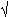DOC HOME SITE MAP MAN PAGES GNU INFO SEARCH PRINT BOOK

# factor(C)

factor -- factor a number

## Syntax

factor [ number ]

## Description

When factor is invoked without an argument, it waits for a number to be typed in. If you type in a positive number less than 2^46 (about 7.2×10^13) it will factor the number and print its prime factors; each one is printed the proper number of times. Then it waits for another number. It exits if it encounters a zero or any non-numeric character.

If factor is invoked with an argument, it factors the number as above and then exits.

The time it takes to factor a number, n, is proportional ton. It usually takes longer to factor a prime or the square of a prime, than to factor other numbers.

## Diagnostics

factor returns an error message if the supplied input value is greater than 2^46 or is not an integer number.